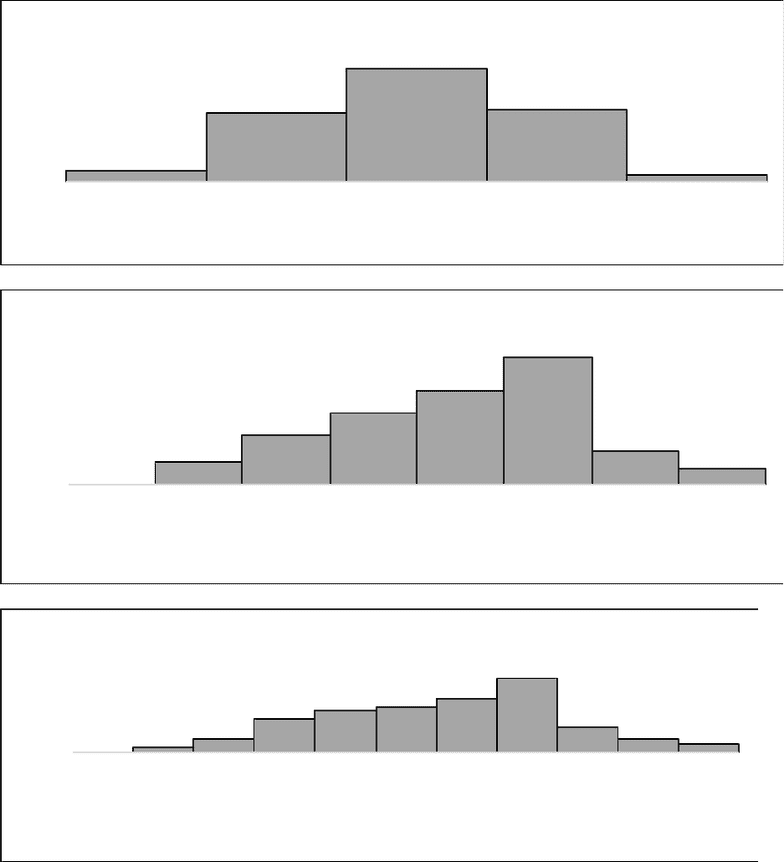# STAT 3010 Lecture Notes - Lecture 7: Histogram, Box Plot, Bar Chart

24 views3 pages
School
Department
Course
Professor3
19
31
20
2
0
5
10
15
20
25
30
35
< = 20
20 < x < = 40
40 < x < = 60
60 < x < = 80
> = 90
Frequency
Monthly Revenue (in Dollars)
Figure 4.1: Histogram of Mothly Revenue (n=75)
0
4
9
13
17
23
63
0
5
10
15
20
25
< = 11
11 < x < = 22
22 < x < = 33
33 < x < = 44
44 < x < = 55
55 < x < = 66
66 < x < = 77
> = 77
Frequency
Monthly Revenue (in Dollars)
Figure 4.2: Histogram of Mothly Revenue (n=75)
013810 11 13 18
632
0
5
10
15
20
< = 8
8 < x < = 16
16 < x < = 32
32 < x < = 40
40 < x < = 48
48 < x < = 56
56 < x < = 64
64 < x < = 72
72 < x < = 80
80 < x < = 88
> = 88
Frequency
Monthly Revenue (in Dollars)
Figure 4.3: Histogram of Monthly Revenue (n=75)
Unlock document

This preview shows page 1 of the document.
Unlock all 3 pages and 3 million more documents.